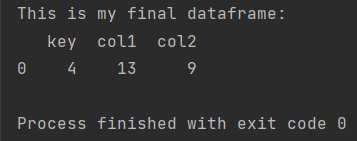Pandas How To Uncategorized How to join two dataframes on condition

# How to join two dataframes on condition

In this post I show how to join two dataframes on a condition in Pandas Python library.

You can join two pandas DataFrames based on a condition by creating a boolean mask that selects the rows to be included in the join and then using the merge method to combine the DataFrames based on this mask.

## How to join two dataframes on condition

Here’s an example of how to perform a join on two DataFrames where the join should include only rows where the value of col1 in df1 is greater than the value of col2 in df2:

```import pandas as pd

my_df1 = pd.DataFrame({'key': [1, 2, 3, 4, 5], 'col1': [15, 7, 3, 13, 2]})
my_df2 = pd.DataFrame({'key': [2, 4, 6, 8, 10], 'col2': [8, 9, 4, 12, 1]})

print(f'This is my final dataframe: \n{my_final_df}')

```In this example, a boolean mask is created using the expression df1[‘col1’] > df2[‘col2’], which returns True for rows where the value of col1 in df1 is greater than the value of col2 in df2. This mask is then used to select the rows from df1 that should be included in the join. Finally, the merge method is used to perform an inner join between the selected rows from df1 and all the rows from df2, based on the key column.

The result of the join will be a new DataFrame that contains only the rows where the value of col1 in df1 is greater than the value of col2 in df2. In this example, the result will have two columns: key and col1, col2.

Tags:

## How to replace values in a columnHow to replace values in a column

Working with data is not easy. Not only you have to prepare, clean and interpret them, you also often have to correct the data. This is a tutorial on how

## How to turn an array into a dataframeHow to turn an array into a dataframe

You can convert a NumPy array to a Pandas DataFrame using the pd.DataFrame constructor: